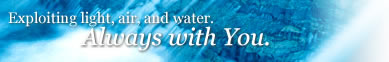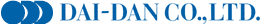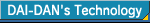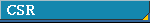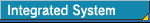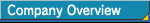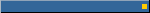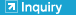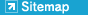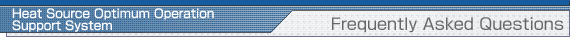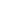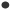OverviewIntroduction ConditionsIntroduction ExamplesFrequently Asked QuestionsIntroduction AccomplishmentsHeat Source Optimum Operation Support System: Frequently Asked Questions
Q: To what does this “Heat Source Optimum Operation Support System” give support?

This System provides an optimum operation program for the heat source equipment to operators conducting the ON-OFF control of heat source equipment and decision-makers of the operation policy, on the air conditioning heat source with large-sized, complicated configuration of equipment.

Q: How does this System determine the optimum operation pattern?
 This System adopts a technique referred to as mathematical programming, and determines the most reasonable combination of equipment that is able to satisfy heat loads demanded at each time.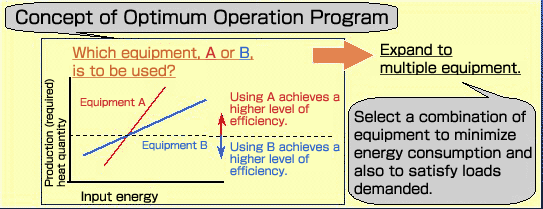Plant model used for the optimized calculations is, so to speak, a group of simultaneous equations (inequalities). (In terms of performance characteristic of individual equipment, the plant’s components are represented as a group of linear equations.) Using this plant model, the conditions of individual equipment are determined so that a certain demanded load is satisfied and also evaluated values (i.e., operation costs and environmental emission loads in this case) referred to as target functions are minimized. These calculations are called “optimized calculations”. On the example shown in the above diagram, it is translated that the system has Equipment A and Equipment B, thus calculating to use which Equipment, A or B, for the production of less input energy when producing a certain quantity of heat with the Equipment. By expanding this concept to the overall configuration of plant, support data to the optimum operation program is provided.
Q: How does this System operate?
The following operation cycle is conducted.1. Loads required for the following 24 hours are forecast.2. Optimized calculation is conducted according to the load forecast and an operation command is issued by time.3. Heat source operator observes this operation command and operates the equipment.4. While monitoring data on the operation results, the optimum operation system issues another command.

Furthermore, this System enables a simulation on whether or not more optimum operation pattern can be provided against the past operating history.
Q: What is the extent of effect obtained?

The extent of effect varies with the configuration of heat source equipment and the current operating conditions.
Past results show approximately 10% on a simulation basis or a few percentages in comparison of operation costs with actual equipment in operation.